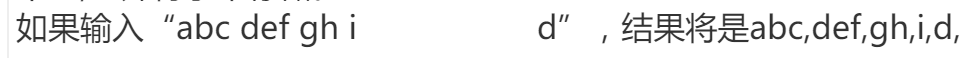C：分离字符串子串问题`````` #include <stdio.h>
#include <string.h>

void DividString(const char *pInputStr, long lInputLen, char *pOutputStr);

int main()
{
char str1, str2, len, i, j;

gets(str1);

len = strlen(str1);

DividString(str1, len, str2);

puts(str2);

getchar();
return 0;
}

void DividString(const char *pInputStr, long lInputLen, char *pOutputStr)
{
int i;

for (i = 0; i < lInputLen; i++)
{
if (pInputStr[i] != ' ')
pOutputStr[i] = pInputStr[i];
else if (pInputStr[i] == ' '&&pInputStr[1 + i] != ' ')
pOutputStr[i] = ',';
else if (pInputStr[i] == ' '&&pInputStr[1 + i] == ' ')
continue;
}
pOutputStr[i++] = ',';
pOutputStr[i] = '\0';
}
``````

2个回答

`````` void DividString(const char *pInputStr, long lInputLen, char *pOutputStr)
{
int i;
bool bCanInputComma = false;
int nCount = 0;

for (i = 0; i < lInputLen; i++)
{
if (pInputStr[i] != ' ')
{
pOutputStr[nCount] = pInputStr[i];
++nCount;
bCanInputComma = true;
}
else if (pInputStr[i] == ' ' && bCanInputComma == true)
{
pOutputStr[nCount] = ',';
++nCount;
bCanInputComma = false;
}
}

pOutputStr[nCount] = ',';
pOutputStr[nCount + 1] = '\0';
}
``````zcmerlin 嗯嗯，我再想一想，谢谢！
3 年多之前 回复AlbertS 回复AlbertS: 重点在于碰到多余的空格时，原串的索引要++，而结果串的索引不变
3 年多之前 回复AlbertS 回复zcmerlin: 你的函数在思考的过程中就有一点问题，当你只用一个变量i来作为索引赋值时，就已经假定原串和结果串是相等长度的，这样就只能把一个空格换成逗号了，然后就出问题了，实际情况应该是结果的字符串可能比原来的字符串短好多，所以需要一个新的变量来记录索引
3 年多之前 回复zcmerlin 嗯，谢谢，用你的函数插断点看了一遍明白了，我还想问一下我写的函数是不是逻辑有问题，可以指点一下吗
3 年多之前 回复

bCanInputComma表示次数能不能输入逗号
nCount表示输出结果的长度Problem Description Everybody knows the number is saved with the binary string in the computer. Now, their have N (1 <= N <= 1000) binary strings I tell you, your task is tell me what is the most binary substring with K (1 <= K <= L) characters in the strings I give you. The length of each string is L (1 <= L <= 60). Output times of the most appearing binary substring. Input Each line will contain three numbers N, L, K. Following N lines, represent N binary strings. Output One answer one line. Sample Input 2 6 3 101011 110101 2 6 4 101011 110101 Sample Output 4 2

java字符串中求字串的个数。求大神指点。谢谢

package z_1; public class Z_4 { /* * 计算某个字符串在另一个字符串中出现的次数 */ public static void main(String[] args) { // TODO Auto-generated method stub String s="kkaaskkadidkkddspqlkk"; getCount(s,"kk"); sop(getCount(s,"kk")); } private static int getCount(String s, String string) { // TODO Auto-generated method stub int count=0; int index=0; while((index=s.indexOf(string))!=-1) { sop("s="+s); s=s.substring(index+string.length()); count++; } return count; } private static void sop(String str) { // TODO Auto-generated method stub System.out.println(str); } }

Problem Description Given a string S and two integers L and M, we consider a substring of S as “recoverable” if and only if (i) It is of length M*L; (ii) It can be constructed by concatenating M “diversified” substrings of S, where each of these substrings has length L; two strings are considered as “diversified” if they don’t have the same character for every position. Two substrings of S are considered as “different” if they are cut from different part of S. For example, string "aa" has 3 different substrings "aa", "a" and "a". Your task is to calculate the number of different “recoverable” substrings of S. Input The input contains multiple test cases, proceeding to the End of File. The first line of each test case has two space-separated integers M and L. The second ine of each test case has a string S, which consists of only lowercase letters. The length of S is not larger than 10^5, and 1 ≤ M * L ≤ the length of S. Output For each test case, output the answer in a single line. Sample Input 3 3 abcabcbcaabc Sample Output 2

python正则替换某2个字符串中任意字符串

Python如何迭代字符串

java同时查找多个字符在同一字符串中的位置

linux shell提取字串中的字串

<div class="post-text" itemprop="text"> <p>I'm trying to read an entire line from the console (including whitespace), then process it. Using bufio.ReadString, the newline character is read together with the input, so I came up with the following code to trim the newline character:</p> <pre><code>input,_:=src.ReadString(' ') inputFmt:=input[0:len(input)-2]+"" //Need to manually add end of string </code></pre> <p>Is there a more idiomatic way to do this? That is, is there already a library that takes care of the ending null byte when extracting substrings for you?</p> <p>(Yes, I know there is already a way to read a line without the newline character in <a href="https://stackoverflow.com/questions/6141604/go-readline-string">go readline -&gt; string</a> but I'm looking more for elegant string manipulation.)</p> </div>

Substring 字符串的子串问题

Problem Description Give you two number a, b. Output the smallest number that can be added to a to contain the number b as a substring. Input The first line contains a single positive integer T( T <= 500 ), indicates the number of test cases. For each test case: Two number a,b; 0 <= a <= 10^100, 0 <= b <= 10^7 Output For each test case: output the case number as shown and the smallest number that satisfy the description. Sample Input 2 9 1 9 2 Sample Output Case #1: 1 Case #2: 3

Problem Description Everybody knows the number is saved with the binary string in the computer. Now, their have N (1 <= N <= 1000) binary strings I tell you, your task is tell me what is the most binary substring with K (1 <= K <= L) characters in the strings I give you. The length of each string is L (1 <= L <= 60). Output times of the most appearing binary substring. Input Each line will contain three numbers N, L, K. Following N lines, represent N binary strings. Output One answer one line. Sample Input 2 6 3 101011 110101 2 6 4 101011 110101 Sample Output 4 2

C/C++ 统计回文子串······

# 字符位置统计（使用指针) ## 【问题描述】 编写一个程序，输入一个字符串str(长度不超过30)和一个字符ch，通过指针查找、统计字符串str中字符ch出现的位置及次数（区分大小写）。画出流程图。 ## 【输入形式】 输入一个字符串str，以及一个字符ch ## 【输出形式】 若ch在str中存在，输出位置，位置之间以空格分隔 若ch在str中不存在，输出NULL 输出后面无换行符 ## 【样例输入1】 Shanghaijiaotongdaxue h ## 【样例输出1】 1 5 2 ## 【样例输入2】 Helloworld @ 【样例输出2】 NULL

KMP算法的next函数怎么理解?

springboot+jwt实现token登陆权限认证

MySQL数据库面试题（2020最新版）

2020阿里全球数学大赛：3万名高手、4道题、2天2夜未交卷

HashMap底层实现原理，红黑树，B+树，B树的结构原理 Spring的AOP和IOC是什么？它们常见的使用场景有哪些？Spring事务，事务的属性，传播行为，数据库隔离级别 Spring和SpringMVC，MyBatis以及SpringBoot的注解分别有哪些？SpringMVC的工作原理，SpringBoot框架的优点，MyBatis框架的优点 SpringCould组件有哪些，他们...

Python爬虫，高清美图我全都要（彼岸桌面壁纸）

Java岗开发3年，公司临时抽查算法，离职后这几题我记一辈子